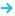Author: Alison Heyerdahl
Published: August 18, 2023Editor: Chris Cammack
Updated: August 21, 2023

A good risk-to-reward ratio helps ensure that you are consistently profitable in the long term. In this video, we cover how to calculate your risk-reward ratio, provide plenty of examples of how risk and reward can inform your trades, and how to determine the right risk-reward ratio for your trading style.

Note that this video is for intermediate or more serious beginner traders who have a grasp of the basics of trading and understand how to manage their risk through stop-losses and take profits.

Transcript

Hello, I’m Alison from FxScouts. In this video, we will discuss how to calculate your risk and reward ratio, understand its significance, and why maintaining a favourable risk-reward ratio is pivotal for long-term profitability.

What is Risk and Reward?

• Risk: The potential amount of money you could lose by holding a certain position.
• Reward: The potential amount of money you could gain by holding that same position.

Understanding the Risk-Reward Ratio

The risk-reward ratio represents the potential loss (risk) of a position compared to the potential gain (reward). A good ratio often sees the reward exceeding the risk.

• Example of a Good Ratio: A risk-reward ratio of 1:3 means that for every dollar risked, there is a potential gain of three dollars.
• Example of a Poor Ratio: A risk-reward ratio of 2:1 means risking twice the amount of potential reward.

Real-World Scenarios

• Good Risk-Reward Setup:
• A trader sets a stop-loss order 50 pips below the entry price and a take-profit order 100 pips above it. The trader could potentially gain double what they risk.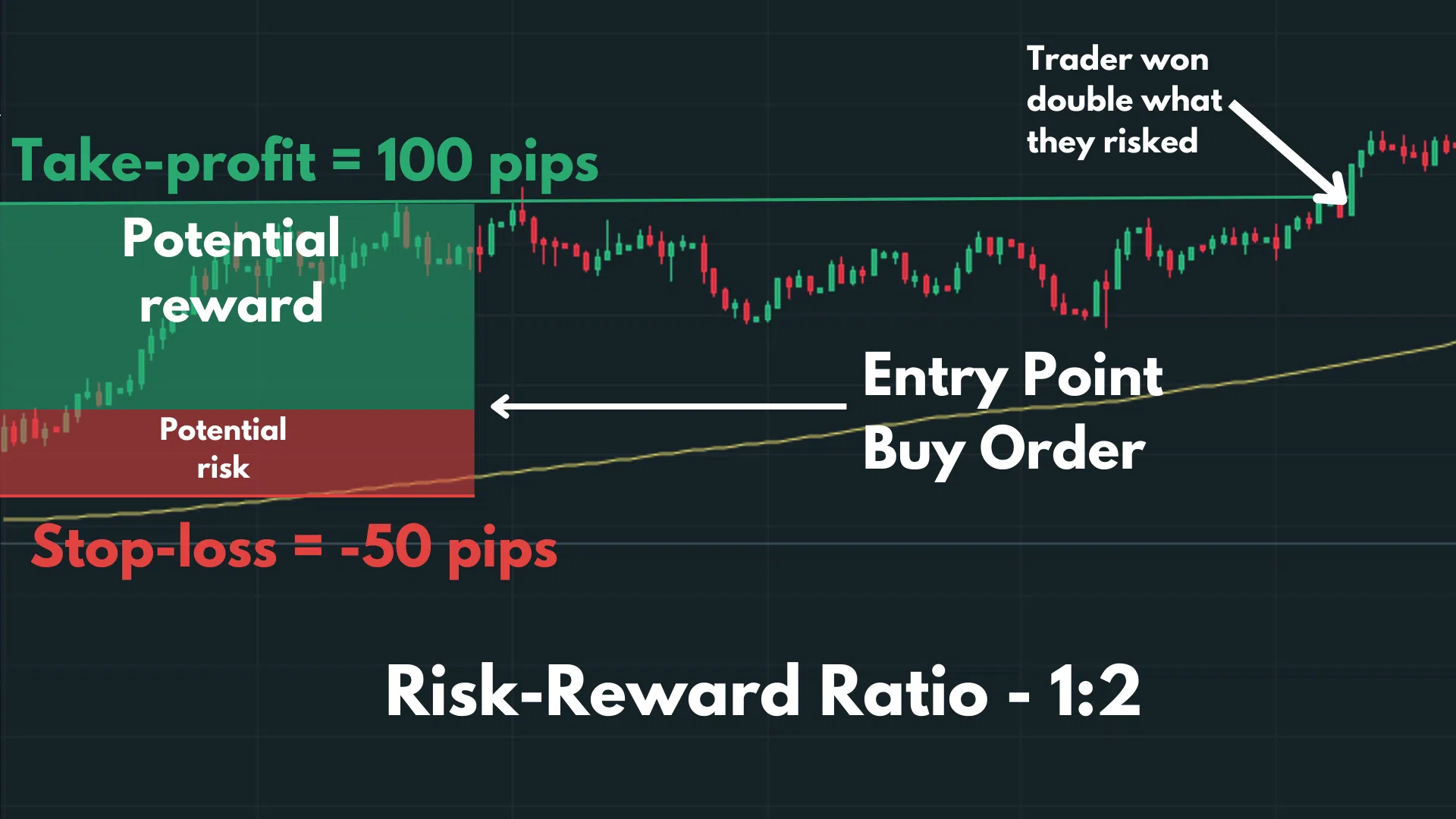• Poor Risk-Reward Setup:
• A trader sets a stop-loss order 100 pips below the entry price and a take-profit order just 50 pips above it. Here, the potential loss is twice the potential gain, making it harder for consistent profitability.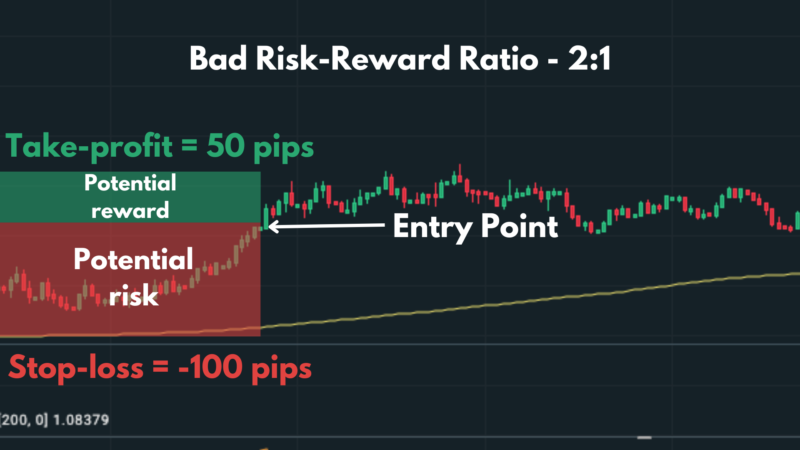Effect of Risk-Reward on Profitability

Even with a win rate of only 50%, a favourable risk-reward ratio can yield positive outcomes.

For instance: For ten trades, alternating between losses of \$1,000 and gains of \$3,000, the total loss would be \$5,000 and the total gain \$15,000, leaving a net profit of \$10,000.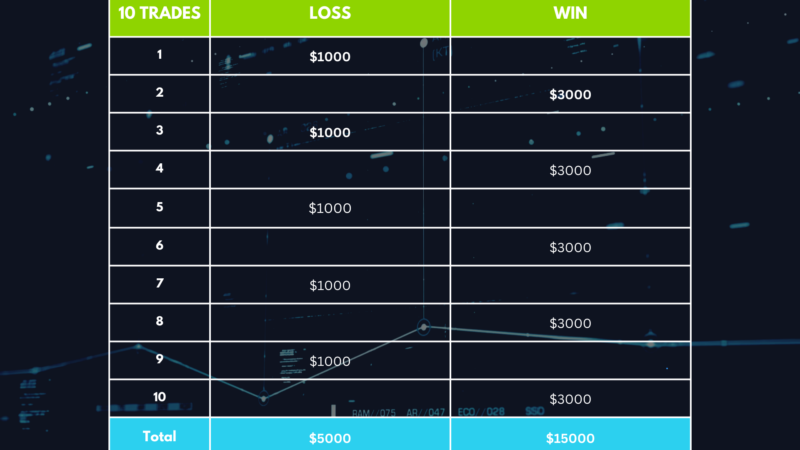Factors Influencing Risk-Reward Ratios

• Experience level
• Market volatility

More experienced traders might opt for a 1:1 or 1:2 ratio, while novices might prefer ratios like 1:3 or 1:4.

1:1 Risk-Reward Ratio

This is favoured by daring or advanced traders. They risk the same amount of capital as they stand to gain. This strategy can result in doubling capital or losing it entirely. Emotions can influence decisions, affecting position sizing and timing.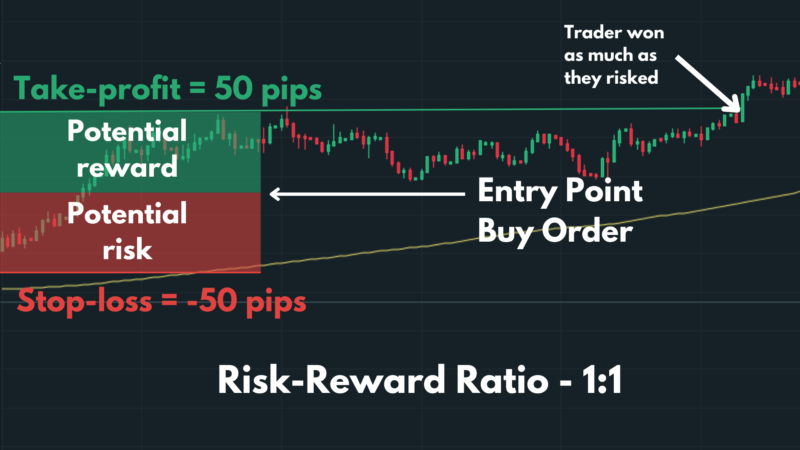Calculating Risk-Reward Ratio

To determine the ratio:

• Measure potential loss (difference between entry and stop-loss).
• Measure potential gain (difference between entry and take-profit).

Formula: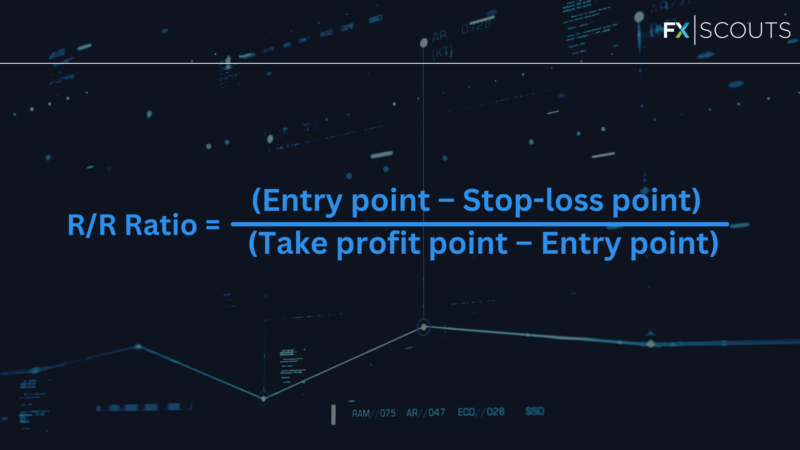It’s crucial to set realistic stop losses and take profits, considering factors like support and resistance levels.

Example Calculations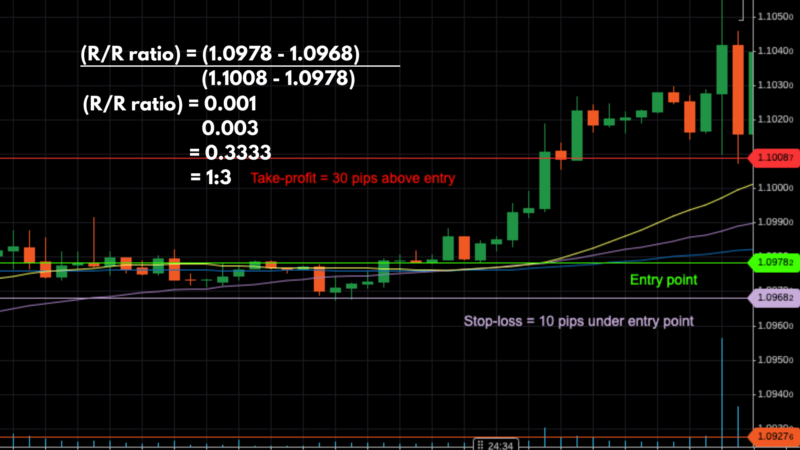Entry: 1.0978, Stop-loss: 1.0968, Take-profit: 1.1008
Using the formula, the risk-reward ratio is 1:3.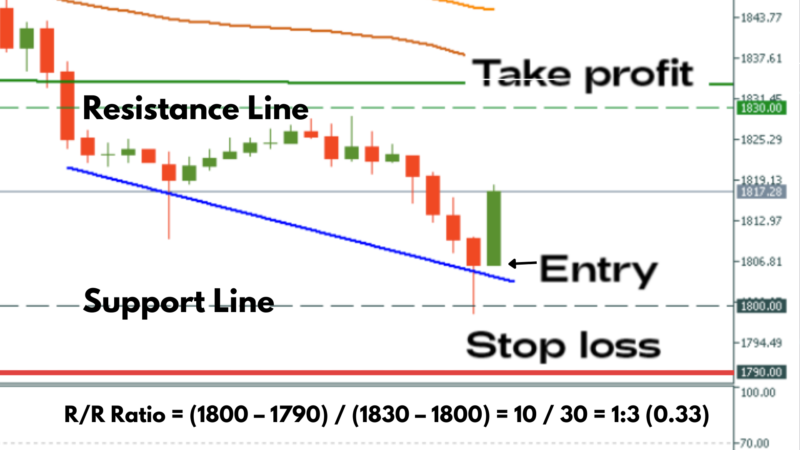Entry: \$1800, Stop-loss: \$1790, Take-profit: \$1830
The risk-reward ratio is again 1:3.

Note: Consider broker costs, spreads, and commissions in calculations.

Summary

Risk-reward ratios need adjustments based on the following:

Conclusion

Thank you for watching. For any questions on risk-reward ratios or other trading topics, contact us at [email protected]

Disclaimer: This transcript summary was created with AI assistance.

## Others Also Visit

Scroll for more details